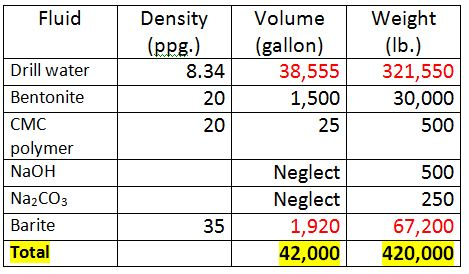# Determine Quantity of Material Used in Mixing a Simple Drilling Mud

This is a simple example to demonstrate how to determine quantity of material required in a simple mixing system. Let’s learn from the example below.

The objective is to build mud which has a weight of 11.0 ppg with 1,000 bbl.

The mud recipe is shown below;

• 30 ppb bentonite
• 5 ppb CMC polymer
• 5 ppb caustic soda (NaOH)
• 25 ppb Na2CO3
• Weighting Material is barite.

The following are important data of water and chemical used for this calculation.

Base fluid = fresh water

Specific gravity of water = 1

Specific gravity of barite = 4.2

Specific gravity of bentonite = 2.4

Specific gravity of CMC polymer = 2.4

Fresh water weight = 8.34 ppg

NaOH and Na3CO3 have negligible volume because it is dissolvable.

What is quantity of material required to meet the mud specification?

What is the final volume?

Weight of chemical (ppb) is based on the volume of drilling mud.

Weight of chemical required as per a mud formula.

Bentonite (30 ppb) = 30 × 1,000= 30,000 lb.

CMC polymer (0.5 ppb) = 0.5 × 1,000 =500 lb.

NaOH (0.5 ppb) = 0.5 × 1,000 =500 lb.

Na2CO3 (0.25 ppb) = 0.25 × 1,000 =250 lb.

Density of material is listed below;

Water = 8.34 ppg.

Barite = 4.2 × 8.34 = 35.0 ppg.

Bentonite = 2.4 × 8.34 = 20.0 ppg.

CMC polymer = 2.4 × 8.34 = 20.0 ppg.

Volume of water in gallon = X gallons

Weight of water (lb.) = volume (gallon) × density of water (ppg)

Weight of water (lb.) = X × 8.34

Volume of barite = Y gallons

Weight of barite (lb.) = volume (gallon) x density of barite (ppg.)

Weight of water (lb.) = Y × 35

Construct the table like this and fill in some data.Volume of bentonite (A) = Weight of bentonite ÷ density of bentonite

Volume of bentonite (A) = 30,000 ÷ 20 = 1,500 gallon

Volume of CMC Polymer (B) = Weight of CMC Polymer ÷ density of CMC Polymer

Volume of CMC Polymer (B) = 500 ÷ 20 = 25 gallon

Volume of NaOH and Na3CO3 will not be considered as they dissolve and have very little effect on volume. They will contribute to the weight of fluid.

The table will be like this.The planed mud weight is 10 ppg, so we can write the relationship like this:

Total Weight (lb.) ÷ Total Volume (gallon) = 10 (ppg)

Total Weight (lb) = 8.34X + 30,000+500+500+250+ 35Y

Total Weight (lb) = 31,250 + 8.34X + 35Y

Total Volume (gallon) = 1,500 + 25 + X + Y

Total Volume (gallon) = 1,525 + X + Y

Therefore;

(31,250 + 8.34X + 35Y) ÷ (1,525 + X + Y) = 10 — Equation 1

Total Volume required = 1,000 bbl = 1,000 × 42 = 42,000 gallon

Note: 1 bbl = 42 US gallon.

Therefore;

42,000 = 1,525 + X + Y — Equation 2

From Equation 2,

X = 40,475 – Y — Equation 3

Substitute from Equation 3 in Equation 1

(31,250 + 8.34X + 35Y) ÷ (1,525 + X + Y) = 10

(31,250 + 8.34X + 35Y) = 10 × (1,525 + X + Y)

(31,250 + 8.34×(40,475 – Y)+ 35Y) = 10 × (1,525 + 40,475 – Y + Y)

Solve Y

Y = 1,920

Substitute Y in Equation 3 to solve X

X = 38,555

The final table is shown below.Summary

In order to build simple water based mud system as per the mud plan, the list of material weight required is shown below;

• Drill water = 321,550 lb. (38,555 gallon)
• Bentonite = 30,000 lb.
• CMC polymer = 500 lb.
• NaOH = 500 lb.
• Na2CO3 = 250 lb.
• Barite = 67,200 lb.

References

Andy Philips, 2012. So You Want to be a Mud Engineer: An Introduction to Drilling Fluids Technology. Edition. CreateSpace Independent Publishing Platform.

Ryen Caenn, 2011. Composition and Properties of Drilling and Completion Fluids, Sixth Edition. 6 Edition. Gulf Professional Publishing.

Share the joy
Tagged , , , . Bookmark the permalink.